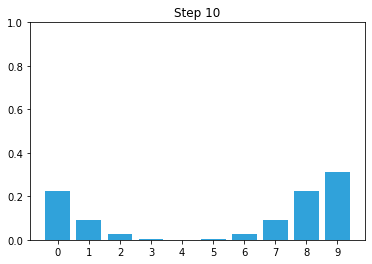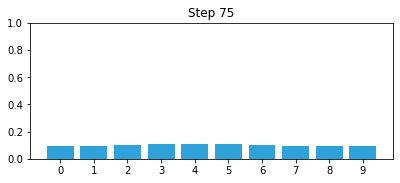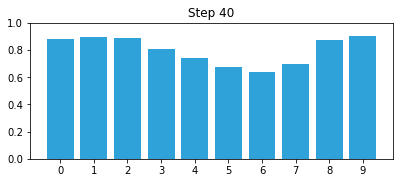# Discrete Bayes Animations¶

In :
import matplotlib.pyplot as plt
import sys
sys.path.insert(0,'..') # allow us to format the book
import book_format
book_format.set_style()

Out:

This notebook creates the animations for the Discrete Bayesian filters chapter. It is not really intended to be a readable part of the book, but of course you are free to look at the source code, and even modify it. However, if you are interested in running your own animations, I'll point you to the examples subdirectory of the book, which contains a number of python scripts that you can run and modify from an IDE or the command line. This module saves the animations to GIF files, which is quite slow and not very interactive.

On Windows you need to install ffmpeg with

\$ conda install -c conda-forge ffmpeg



I don't know what to do for linux or MaxOS.

In :
from matplotlib import animation
import matplotlib.pyplot as plt
import numpy as np
from kf_book.book_plots import bar_plot
%matplotlib inline

# the predict algorithm of the discrete bayesian filter
def predict(pos, move, p_correct, p_under, p_over):
n = len(pos)
result = np.array(pos, dtype=float)
for i in range(n):
result[i] =  \
pos[(i-move) % n]   * p_correct + \
pos[(i-move-1) % n] * p_over + \
pos[(i-move+1) % n] * p_under
return result

def normalize(p):
s = sum(p)
for i in range (len(p)):
p[i] = p[i] / s

# the update algorithm of the discrete bayesian filter
def update(pos, measure, p_hit, p_miss):
q = np.array(pos, dtype=float)
for i in range(len(hallway)):
if hallway[i] == measure:
q[i] = pos[i] * p_hit
else:
q[i] = pos[i] * p_miss
normalize(q)
return q

In :
import matplotlib
# make sure our matplotlibrc has been edited to use imagemagick
print(matplotlib.matplotlib_fname())
matplotlib.rcParams['animation.writer']

C:\Anaconda3\lib\site-packages\matplotlib\mpl-data\matplotlibrc

Out:
'ffmpeg'
In :
from kf_book.gif_animate import animate

pos = [1.0,0,0,0,0,0,0,0,0,0]
def bar_animate(nframe):
global pos
plt.cla()
bar_plot(pos)
plt.title('Step {}'.format(nframe + 1))
pos = predict(pos, 1, .8, .1, .1)

for i in range(10):
bar_animate(i)

fig = plt.figure(figsize=(6.5, 2.5))
animate('02_no_info.gif', bar_animate, fig=fig, frames=75, interval=75);In :
pos = np.array([.1]*10)
hallway = np.array([1, 1, 0, 0, 0, 0, 0, 0, 1, 0])

def bar_animate(nframe):
global pos
#if nframe == 0:
#   return

bar_plot(pos, ylim=(0,1.0))
plt.title('Step {}'.format(nframe + 1))
if nframe % 2 == 0:
pos = predict(pos, 1, .9, .05, .05)
else:
x = int((nframe/2) % len(hallway))
z = hallway[x]
pos = update(pos, z, .9, .2)

fig = plt.figure(figsize=(6.5, 2.5))
animate('02_simulate.gif', bar_animate, fig=fig, frames=40, interval=85);